Pythagoras Theorem and its Converse – Triangles | Class 10 Maths

• Difficulty Level : Easy
• Last Updated : 01 Sep, 2021

The Theorem of Pythagoras is a well-known theorem, which is also a very old one. Pythagoras theorem provides us with the relationship between the sides in a right-angled triangle. A right triangle consists of two legs and a hypotenuse. The two legs meet at a 90° angle and the hypotenuse is the longest side of the right triangle and is the side opposite the right angle.

In a right triangle, the square of the hypotenuse is equal to the sum of the squares of the other two sides.

Formula for Pythagoras Theorem

Consider the triangle given below Where “a” is the perpendicular side, “b” is the base, and “c” is the hypotenuse side then according to the definition, the Pythagoras Theorem formula is given as: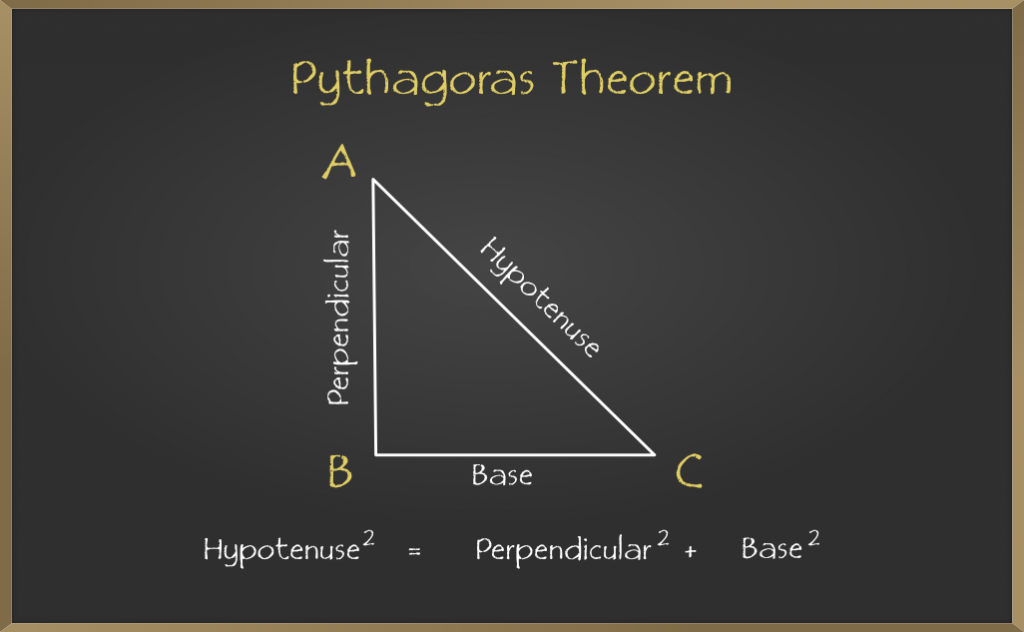Proof for Pythagoras Theorem

Let’s see the traditional way to prove Pythagoras theorem formula which says that the area of the square on the hypotenuse is equal to the sum of the areas of the squares on the two shorter sides. The below figure shows squares drawn on every side.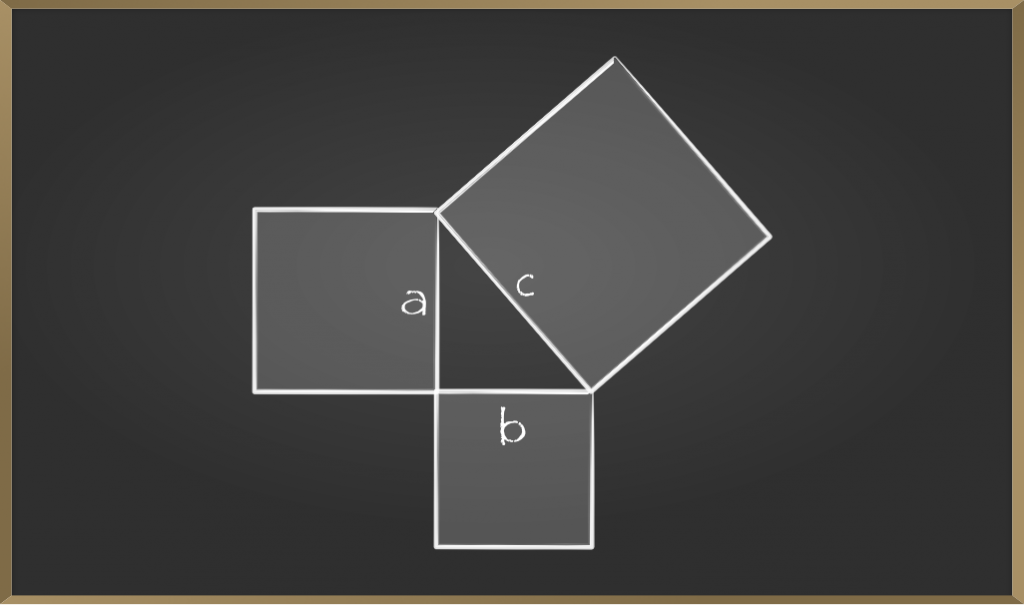Consider three squares of sides a, b, c mounted on the three sides of a triangle A, B, and C respectively.

By Pythagoras Theorem:

Area of square A + Area of square B = Area of square C

which is nothing but,

a2 + b2 = c2

Now let’s solve some problems to get understand the concept better.

Sample Problems on Pythagoras Theorem

Problem 1: In the below given right triangle find the value of y.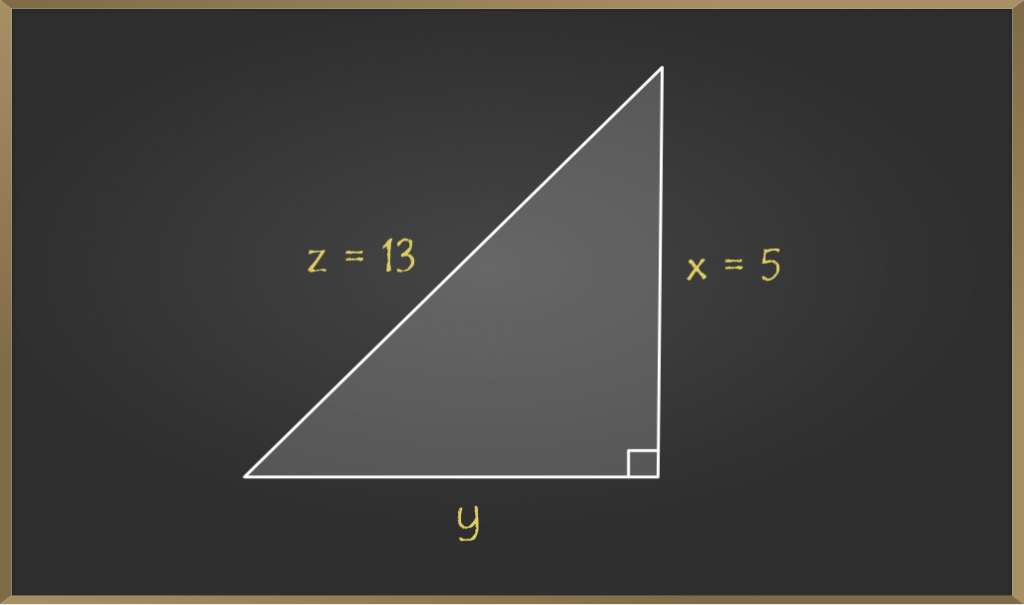Solution:

By the statement of the Pythagoras theorem we get,

=> z2 = x2 + y2

Now, substituting the values directly we get,

=> 132 = 52 + y2

=> 169 = 25 + y2

=> y2 = 144

=> y = √144 = 12

Problem 2: Given a rectangle with length as 4 cm and breadth as 3 cm. Find the length of the diagonal of the rectangle?

Solution: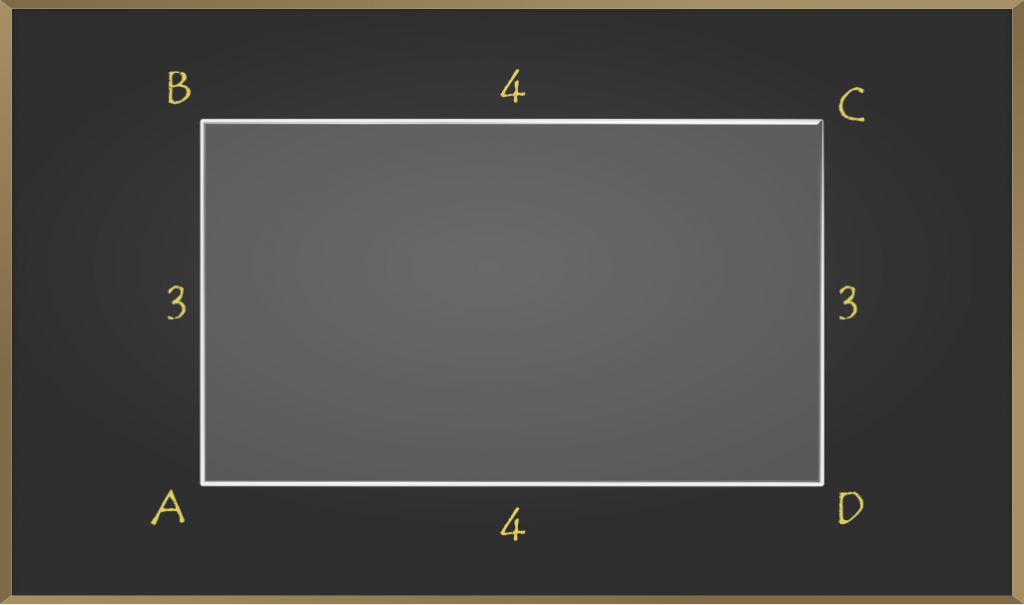In the above diagram length of the rectangle is 4 cm and the width is 3 cm.

Now we have to find the distance between point A to point C or point B to point D. Both give us the same answer because opposite sides are of the same length i.e AC = BD

Now let’s find the distance between points A and C by drawing an imaginary line.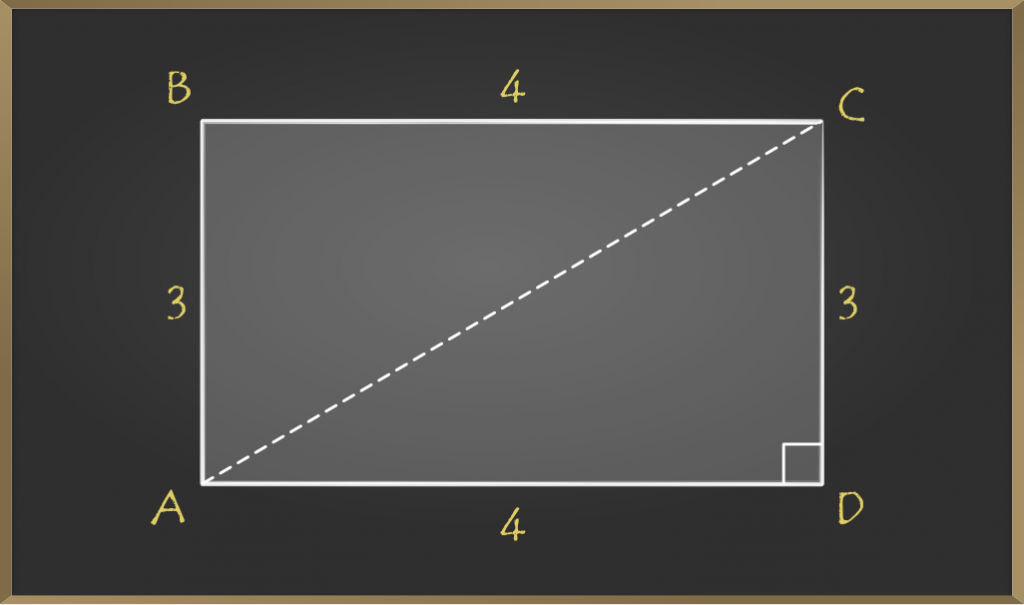Now triangle ACD is a right triangle.

So by the statement of Pythagoras theorem,

=> AC2 = AD2 + CD2

=> AC2 = 42 + 32

=> AC2 = 25

=> AC = √25 = 5

Therefore length of the diagonal of given rectangle is 5 cm.

Converse of Pythagoras theorem

The converse of the Pythagoras theorem is very similar to Pythagoras theorem. To understand this theorem you should think from the reverse of Pythagoras theorem.

If the square of the length of the longest side of a triangle is equal to the sum of the squares of the other two sides, then the triangle is a right triangle.

Formula and Proof of Converse Pythagoras Theorem

The formula will be the same as it is just converse of the Pythagoras theorem. According to the statement we need to prove that the condition occurs then the triangle should be the right triangle, for that we need to prove that the opposite angle of the longest side should be 90°.

If there is a triangle with length a, b and c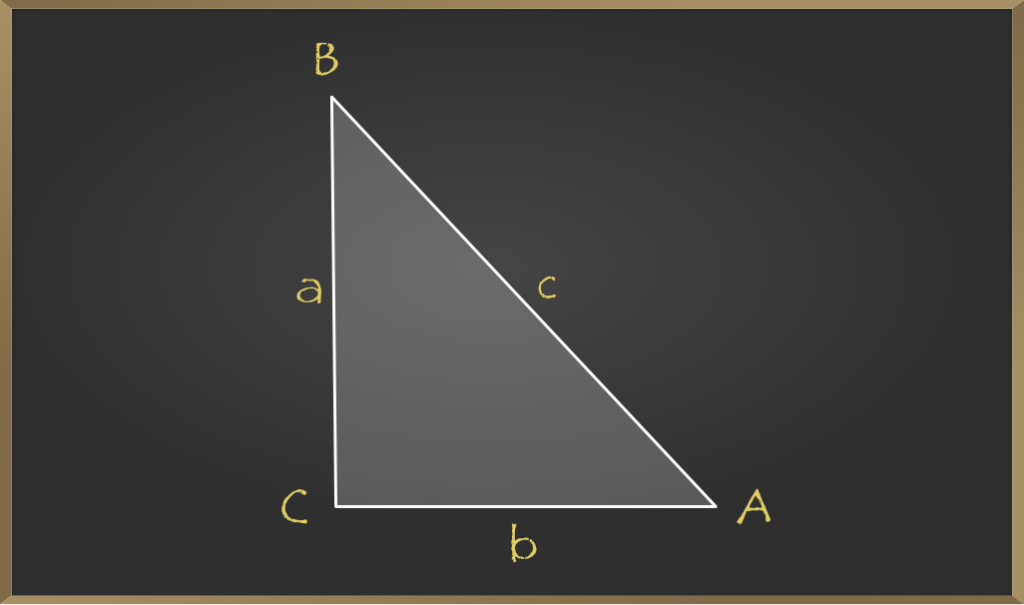We assume that it satisfies c2 = a2 + b2 and by looking into the diagram we can tell that ∠C = 90° but to prove it we require another triangle △EGF, such as AC = EG = b and BC = FG = a.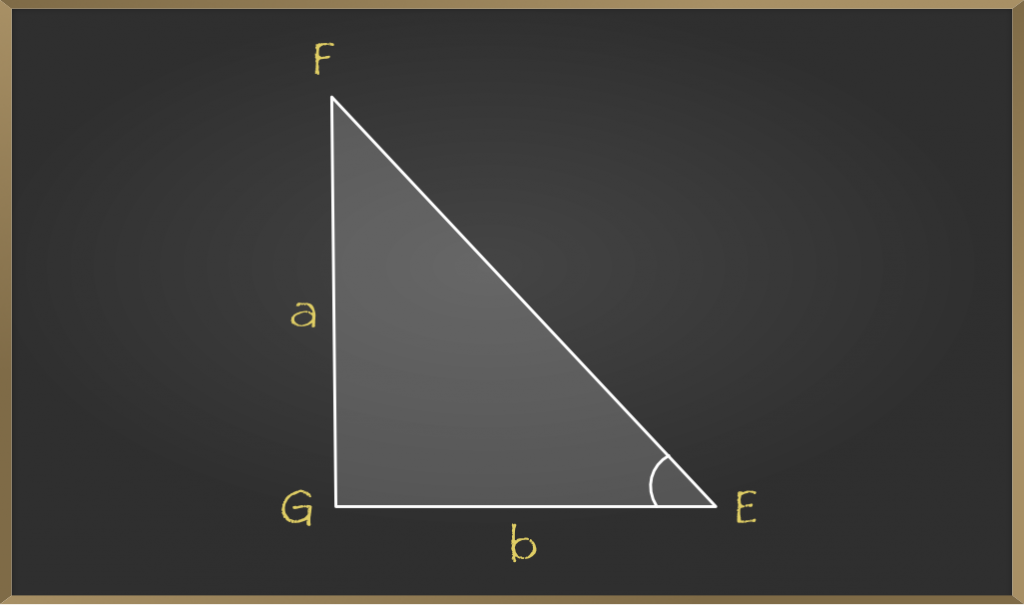In △EGF, by Pythagoras Theorem:

=> EF2 = EG2 + FG22 = b2 + a2 …………(1)

In △ABC, by Pythagoras Theorem:

=> AB2 = AC2 + BC2 = b2 + a2 …………(2)

From equation (1) and (2), we have;

=> EF2 = AB2

=> EF = AB

=> △ ACB ≅ △EGF (By SSS postulate)

=> ∠G is right angle

Thus, △EGF is a right triangle.

Hence, we can say that the converse of the Pythagorean theorem also holds.

Sample Problems on Converse of Pythagoras Theorem

Problem 1: The sides of a triangle are 5, 12, and 13. Check whether the given triangle is a right triangle or not?

Solution:

Given,

=> a = 5

=> b = 12

=> c = 13

By using the converse of Pythagorean Theorem,

=> a2+b2 = c2

Substitute the given values in the above equation,

=> 132 = 52+122

=> 169 = 25 + 144

=> 169 = 169

So, the given lengths satisfy the above condition.

Therefore, the given triangle is a right triangle.

Problem 2: The side of a triangle is of length 9 cm, 11 cm, and 6 cm. Is this triangle a right triangle? If so, which side is the hypotenuse?

Solution:

We know that hypotenuse is the longest side. If 9 cm, 11 cm, and 6 cm are the lengths of the angled triangle, then 11 cm will be the hypotenuse.

Using the converse of Pythagoras theorem, we get

=> (11)2 = (9)2 + (6)2

=> 121 = 81 + 36

=> 121 ≠ 117

Since, both the sides are not equal therefore 9 cm, 11 cm and 6 cm are not the side of the right-angled triangle.

My Personal Notes arrow_drop_up